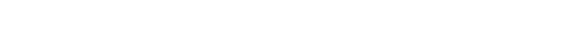Today’s GMAT algebra problem and case study comes from the OG 12th Edition, Problem Solving Practice Problem 173.

Here is the problem as it appears in the Official Guide:

173) Which of the following describes all values for which $$1-{x^2}$$>$$=0$$

OK, this is a quadratic inequality, a relatively advanced math topic for the GMAT, one with a relatively low probability of appearing when you take the GMAT. Nevertheless, if you are ambitious about doing well on math, this is a good topic to master.

OK, first terminology and the basics:

An inequality —- a mathematical statement in which the “verb” is not an equal sign but rather one of the inequality signs (, =). If the inequality contains a variable, the solution for the variable will be a range. By contrast, when you solve an equation, you typically get one or two discrete values as the solution.

A quadratic —- any equation or inequality in which the variable, the unknown, is squared. Quadratic equations often have two solutions. Quadratic inequalities can have two ranges for the solution.

## Solve the Equation First

Step one for solving a quadratic inequality is to replace the inequality sign with an equal sign and solve the equation.

Here, we get:

$$1-x^2=0$$

Factor:

$$(1+x)(1-x)=0$$

Apply the Zero Product Property

$$(1+x)=0 or (1-x)=0$$

$$x=-1 or x=1$$

If the question were asking us to solve the equation, we would be done. In the solution to a quadratic inequality, the solution to the equation gives you boundary values. Here, x = 1 and x = -1 are boundary values: we know something about the solution could start or stop there, but we don’t know what yet.

## The Segmented Number Line

Now that we have our boundary values from solving the equation, we put them on a number line, dividing the number line into segments or regions:Here, the three regions are represented by three different colors.

## Test Each Region

For a quadratic inequality, it is sufficient to pick a test value from each region and see if it satisfies the inequality. Just picking one value, any value in the region, will work as a representative of that entire region. Here:

For the blue region ($$x$$<$$=-1$$), pick $$x=-2$$. Then

$$1-(-2)^2=1-4=-3$$, which is not greater than or equal to zero. This doesn’t work.

For the red region $$(-1$$<$$=x$$<$$=1)$$, pick $$x=0$$, the easiest possible value. Then

$$1-(0)^2=1-0=1$$, which is greater than or equal to zero. This works.

For the green region $$(1$$<$$=x)$$, pick $$x=3$$. Then

$$1-(3)^2=1-9=-8$$, which is not greater than or equal to zero. This doesn’t work.

At this point, we’re done. In this problem, the only region that works is the red region, so the solution is (E) $$-1$$<$$=x$$<$$=1$$.

## Author

•Mike served as a GMAT Expert at Magoosh, helping create hundreds of lesson videos and practice questions to help guide GMAT students to success. He was also featured as "member of the month" for over two years at GMAT Club. Mike holds an A.B. in Physics (graduating magna cum laude) and an M.T.S. in Religions of the World, both from Harvard. Beyond standardized testing, Mike has over 20 years of both private and public high school teaching experience specializing in math and physics. In his free time, Mike likes smashing foosballs into orbit, and despite having no obvious cranial deficiency, he insists on rooting for the NY Mets. Learn more about the GMAT through Mike's Youtube video explanations and resources like What is a Good GMAT Score? and the GMAT Diagnostic Test.